/

### The generalised Pareto distribution

The generalised Pareto distribution (generalized Pareto distribution) arises in Extreme Value Theory (EVT). If the relevant regularity conditions are satisfied then the tail of a distribution (above some suitably high threshold), i.e. the distribution of ‘threshold exceedances’, tends to a generalized Pareto distribution.

Care is needed with EVT because what we are in effect doing with it is to extrapolate into the tail of the distribution. Extrapolation is an intrinsically imprecise and subjective mathematical activity. We can in effect view the regularity conditions that need to be satisfied if EVT applies as corresponding to requiring that this extrapolation is done in a particular manner.

 Distribution name Generalised Pareto distribution (GPD) Common notation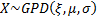Parameters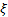= shape parameter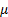= location parameter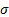= scale parameter (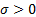) Domain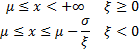Probability density function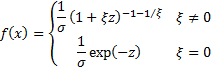where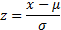Cumulative distribution function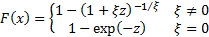Mean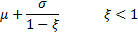Variance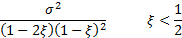Skewness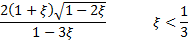(Excess) kurtosis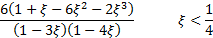Other comments If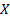is uniformly distributed,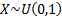then the variable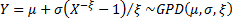.   The mean excess function for a GPD, i.e.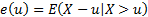takes a particularly simple form which is linear in, i.e.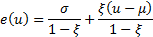Nematrian web functions

Functions relating to the above distribution may be accessed via the Nematrian web function library by using a DistributionName of “generalised pareto”. For details of other supported probability distributions see here.